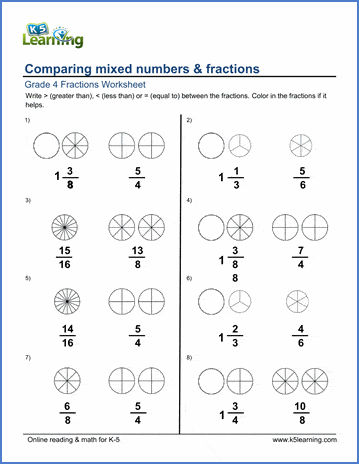# Comparing mixed numbers & fractions

## Worksheets: Comparing mixed numbers & fractions  with pie charts

Below are six versions of our grade 4 fractions worksheet on comparing (less than, greater than, equal to) mixed numbers & proper and improper fractions.  Students can color in the pie charts to illustrate the fractions. These math worksheets are pdf files.## More fractions worksheets

Explore all of our fractions worksheets, from dividing shapes into "equal parts" to multiplying and dividing improper fractions and mixed numbers.

## What is K5?

K5 Learning offers reading and math worksheets, workbooks and an online reading and math program for kids in kindergarten to grade 5.  We help your children build good study habits and excel in school.